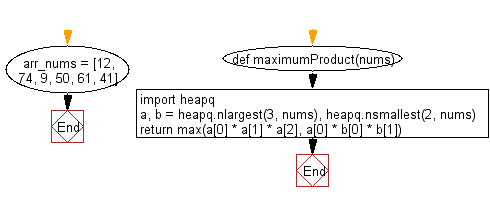﻿ Python heap queue algorithm: Compute maximum product of three numbers of a given array of integers using Heap queue algorithm - w3resource

# Python: Compute maximum product of three numbers of a given array of integers using Heap queue algorithm

## Python heap queue algorithm: Exercise-8 with Solution

Write a Python program to compute the maximum product of three numbers in a given array of integers using the heap queue algorithm.

Sample Solution:

Python Code:

``````def maximumProduct(nums):
import heapq
a, b = heapq.nlargest(3, nums), heapq.nsmallest(2, nums)
return max(a * a * a, a * b * b)
arr_nums = [12, 74, 9, 50, 61, 41]
print("Original array elements:")
print(arr_nums)
print("Maximum product of three numbers of the said array:")
print(maximumProduct(arr_nums))
```
```

Sample Output:

```Original array elements:
[12, 74, 9, 50, 61, 41]
Maximum product of three numbers of the said array:
225700
```

Flowchart:Python Code Editor:

Have another way to solve this solution? Contribute your code (and comments) through Disqus.

What is the difficulty level of this exercise?

Test your Programming skills with w3resource's quiz.

﻿